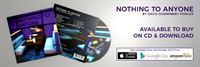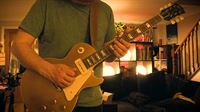# Pixel Noise Geek Stuff

## Create wave files from pictures with VB.NET

Hi everyone,

I thought I'd share the code behind pixel noise here. Some of it was taken from this website, converted to VB.NET and then modified to allow me to generate note sequences rather than just one tone.

To see the videos related to this go to /videos/pixel-noise-music-from-images

This bit is the code that makes wave files:

Imports System.IO

Public Class DDFSound

Public sGroupID As String
' RIFF
Public dwFileLength As UInteger
' total file length minus 8, which is taken up by RIFF
Public sRiffType As String
' always WAVE
''' <summary>
''' Initializes a WaveHeader object with the default values.
''' </summary>
Public Sub New()
dwFileLength = 0
sGroupID = "RIFF"
sRiffType = "WAVE"
End Sub
End Class

Public Class WaveFormatChunk
Public sChunkID As String
' Four bytes: "fmt "
Public dwChunkSize As UInteger
' Length of header in bytes
Public wFormatTag As UShort
' 1 (MS PCM)
Public wChannels As UShort
' Number of channels
Public dwSamplesPerSec As UInteger
' Frequency of the audio in Hz... 44100
Public dwAvgBytesPerSec As UInteger
' for estimating RAM allocation
Public wBlockAlign As UShort
' sample frame size, in bytes
Public wBitsPerSample As UShort
' bits per sample
''' <summary>
''' Initializes a format chunk with the following properties:
''' Sample rate: 44100 Hz
''' Channels: Stereo
''' Bit depth: 16-bit
''' </summary>
Public Sub New()
sChunkID = "fmt "
dwChunkSize = 16
wFormatTag = 1
wChannels = 2
dwSamplesPerSec = 44100
wBitsPerSample = 16
wBlockAlign = CUShort(wChannels * (wBitsPerSample / 8))
dwAvgBytesPerSec = dwSamplesPerSec * wBlockAlign
End Sub
End Class

Public Class WaveDataChunk
Public sChunkID As String
' "data"
Public dwChunkSize As UInteger
' Length of header in bytes
Public shortArray As Short()
' 8-bit audio
''' <summary>
''' Initializes a new data chunk with default values.
''' </summary>
Public Sub New()
shortArray = New Short(-1) {}
dwChunkSize = 0
sChunkID = "data"
End Sub
End Class

Public Class WaveGenerator

Private format As WaveFormatChunk
Private data As WaveDataChunk

Public Sub New()
' Init chunks
format = New WaveFormatChunk()
data = New WaveDataChunk()

' Fill the data array with sample data

data.shortArray = New Short(0) {}

End Sub

Public Sub addFreq(freq As Double, amplitude As Integer, length As Double)

' Number of samples = sample rate * channels * bytes per sample
Dim numSamples As UInteger = format.dwSamplesPerSec * format.wChannels * length

If numSamples = 0 Then Exit Sub

' Initialize the 16-bit array
' data.shortArray = New Short(numSamples - 1) {}
Dim l = data.shortArray.Length
ReDim Preserve data.shortArray(l - 1 + numSamples - 1)

'   Dim amplitude As Integer = 32760
' Max amplitude for 16-bit audio
'   Dim freq As Double = 440.0F
' Concert A: 440Hz
' The "angle" used in the function, adjusted for the number of channels and sample rate.
' This value is like the period of the wave.
Dim t As Double = (Math.PI * 2 * freq) / (format.dwSamplesPerSec * format.wChannels)

For i As UInteger = l - 1 To l + numSamples - 3
' Fill with a simple sine wave at max amplitude
For channel As Integer = 0 To format.wChannels - 1
data.shortArray(i + channel) = Convert.ToInt16(amplitude * Math.Sin(t * i))
Next
Next

' Calculate data chunk size in bytes
data.dwChunkSize = CUInt(data.shortArray.Length * (format.wBitsPerSample / 8))

End Sub

Public Sub Save(filePath As String)
' Create a file (it always overwrites)
Dim fileStream As New FileStream(filePath, FileMode.Create)

' Use BinaryWriter to write the bytes to the file
Dim writer As New BinaryWriter(fileStream)

' Write the format chunk
writer.Write(format.sChunkID.ToCharArray())
writer.Write(format.dwChunkSize)
writer.Write(format.wFormatTag)
writer.Write(format.wChannels)
writer.Write(format.dwSamplesPerSec)
writer.Write(format.dwAvgBytesPerSec)
writer.Write(format.wBlockAlign)
writer.Write(format.wBitsPerSample)

' Write the data chunk
writer.Write(data.sChunkID.ToCharArray())
writer.Write(data.dwChunkSize)
For Each dataPoint As Short In data.shortArray
writer.Write(dataPoint)
Next

writer.Seek(4, SeekOrigin.Begin)
Dim filesize As UInteger = CUInt(writer.BaseStream.Length)
writer.Write(filesize)

' Clean up
writer.Close()
fileStream.Close()
End Sub

End Class

Public Enum waveExampleType
ExampleSineWave = 0
End Enum

End Class

And this code generates the waves from the image:

Dim wg As New DDFSound.WaveGenerator()

Dim bmp As New Bitmap("G:\Dave\My Pictures\image.jpg")

For x = 0 To bmp.Width - 1

For y = 0 To bmp.Height - 1

Dim p = bmp.GetPixel(x, y)

Dim amp = p.GetBrightness * 30000

Dim harm = CInt(p.B / (255 / 12))

If harm = 0 Then harm = 12

harm = filterScale(harm)

Dim octaves = 8

Dim freq As Single = (55.0F + (55.0F * (harm / 12))) * (CInt(p.G * (octaves  / 255)) + 1)

Dim length = ((p.R + 1) / 255) * 0.25

Next

Next

bmp.Dispose()

wg.Save("G:\Dave\My Music\Generated\test.wav")

## Latest Blogs## New Album - Nothing To Anyone## My '54 True Historic Gibson Les Paul Goldtop